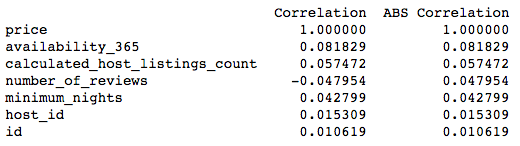# Exploring Data in DataFrames with Python Pandas

## Get DataFrame Columns Names

data.columns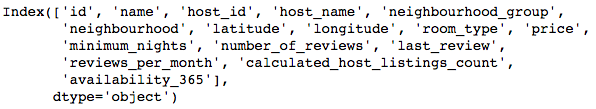Find the data types and non-null rows for each column in a DataFrame:

data.info()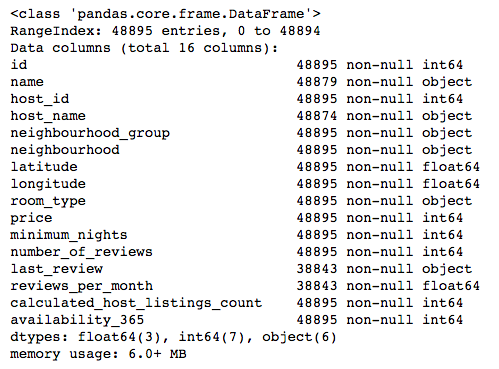## Get Descriptive Statistics for Numerical Columns

View descriptive statisctics including mean, standard deviation and minimum and maximum values for each numerical column in the DataFrame:

data.describe()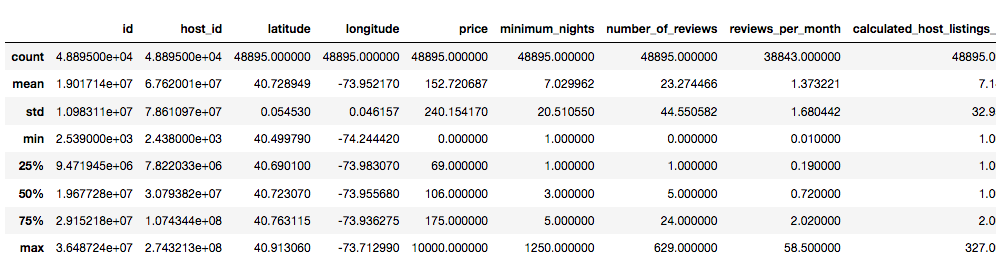## Visualise DataFrame Null Values Using a Heatmap

Display a heatmap showing where light blocks indicate null values in the DataFrame and which columns they belong to on a 10 x 4 plot. Requires Seaborn and Matplotlib to be imported.

plt.figure(figsize=(10,4))
sns.heatmap(data.isnull(),yticklabels=False,cbar=False)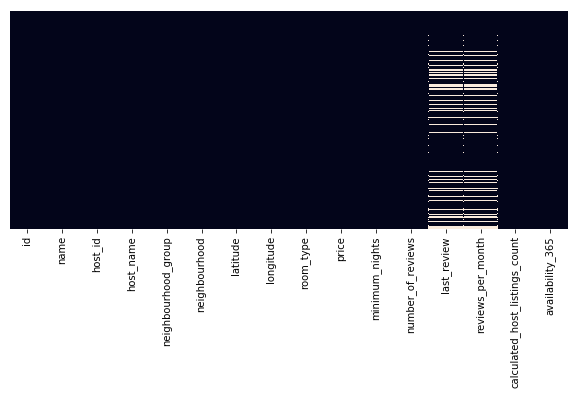## Correlation Heatmap

Display a labelled heatmap showing the correlation values between the numerical columns in the Data DataFrame on a 10 x 8 plot. Requires Seaborn and Matplotlib to be imported.

plt.figure(figsize=(10,8))
sns.heatmap(data.corr(),annot=True)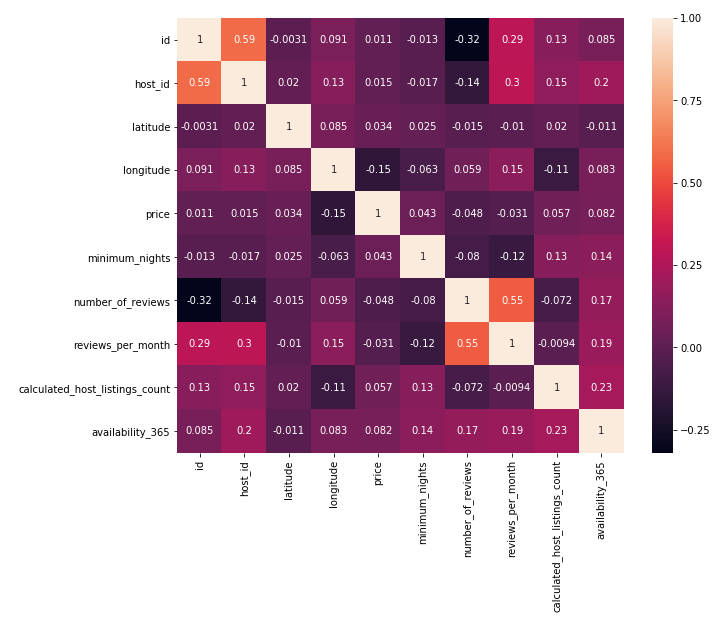## Correlation for a Particular Column

Display the correlation value between the numerical columns in the DataFrame and the 'price' column:

data.corr()['price']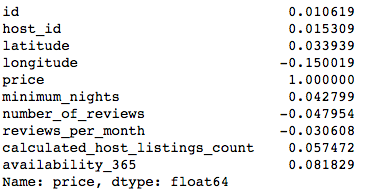## Sorted Correlation for a Particular Column

Display the correlation and absolute column values between the numerical columns in the DataFrame and the 'price' column in order to show the most correlated or inversely correlated columns:

def correlation_table(data,target_column):
data_num = data.select_dtypes(include=['int'])
corr_df = pd.DataFrame(data_num.corrwith(data_num[target_column]),columns=['Correlation']).dropna()
corr_df['ABS Correlation'] = abs(corr_df['Correlation'])
corr_df.sort_values(by=['ABS Correlation'], ascending=False, inplace=True)
print(corr_df)

correlation_table(data, 'price')